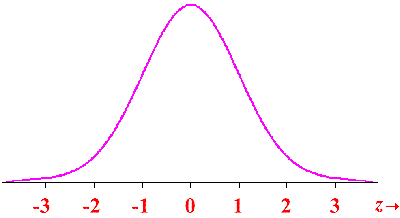# Understanding to normal distribution and standard normal deviation

What is the difference between a normal distribution and standard normal deviation?

A normal distribution with a mean of 0 and a standard deviation of 1 is called a standard normal distribution. The normal distribution is a probability distribution that is symmetric about the mean. In graph form it is a bell-shaped curve.

A Standard Normal Distribution is a normal distribution with mean 0 and standard deviation 1.Areas under this curve can be found using a standard normal table.

The normal random variable of a standard normal distribution is called a standard score or a z-score. Every normal random variable X can be transformed into a z score via the following equation:

𝑧 = (𝑋−μ)/σ

where X is a normal random variable, μ is the mean of X, and σ is the standard deviation of X.

The standard Normal Distribution has a mu (mean) of 0 and a sigma (standard deviation) of 1.
A Normal Distribution has a mean and standard deviation that are equal to those of observations (a dataset).